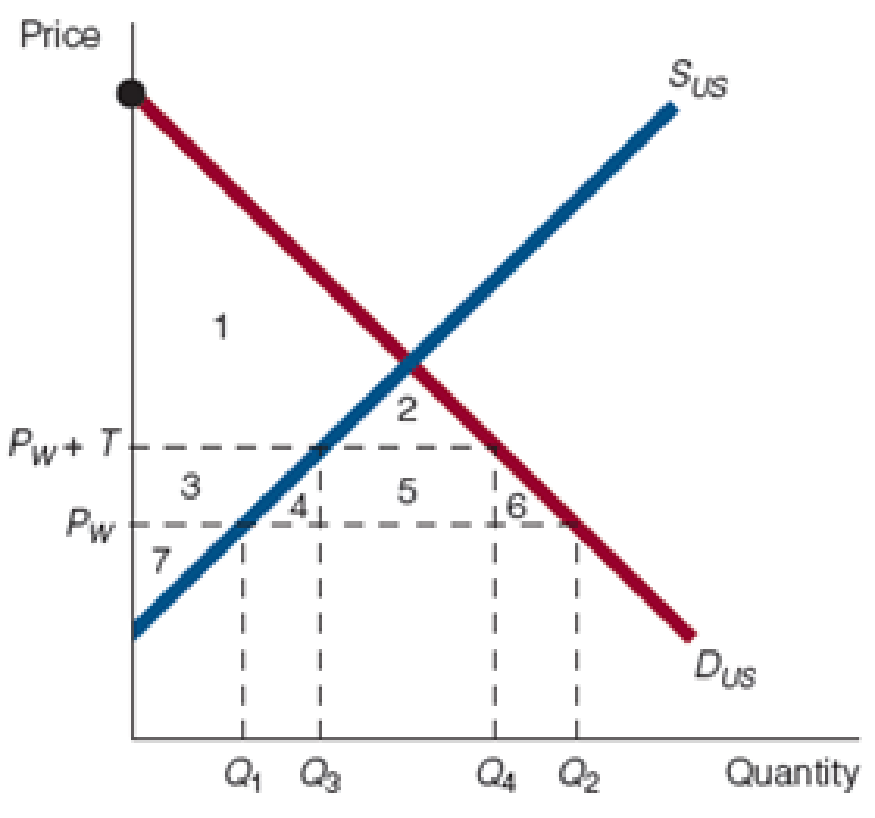Chapter 33, Problem 2WNG

Chapter
Section
Textbook Problem

In the accompanying figure, PW is the world price and PW + T is the world price plus a tariff. Identify the following: a. The level of imports at PW b. The level of imports at PW + T c. The loss in consumers’ surplus as a result of a tariff d. The gain in producers’ surplus as a result of a tariff e. The revenue received as a result of a tariff f. The net loss to society as a result of a tariff g. The net benefit to society of moving from a tariff to no tariff(a)

To determine

The level of imports at ‘Pw’.

Explanation

Figure 1 shows the demand and supply curve of Country U. In this figure, the quantity is measured on the horizontal axis, and price is measured on the vertical axis...

(b)

To determine

The level of import at ‘Pw+T’.

(c)

To determine

The loss in consumer surplus as a result of tariff.

(d)

To determine

The gain in producer surplus as a result of tariff.

(e)

To determine

The revenue received as a result of tariff.

(f)

To determine

The net loss to society as a result of tariff.

(g)

To determine

The net benefit to society moving from tariff to non-tariff.

Still sussing out bartleby?

Check out a sample textbook solution.

See a sample solution

The Solution to Your Study Problems

Bartleby provides explanations to thousands of textbook problems written by our experts, many with advanced degrees!

Get Started

Find more solutions based on key concepts